﻿ Twofish Fields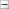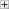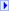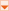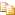The Twofish type exposes the following members.

#Fields

NameDescription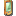BlockSizeValue
Represents the block size, in bits, of the cryptographic operation.
(Inherited from SymmetricAlgorithm.)FeedbackSizeValue
Represents the feedback size, in bits, of the cryptographic operation.
(Inherited from SymmetricAlgorithm.)IVValue
Represents the initialization vector (IV) for the symmetric algorithm.
(Inherited from SymmetricAlgorithm.)KeySizeValue
Represents the size, in bits, of the secret key used by the symmetric algorithm.
(Inherited from SymmetricAlgorithm.)KeyValue
Represents the secret key for the symmetric algorithm.
(Inherited from SymmetricAlgorithm.)LegalBlockSizesValue
Specifies the block sizes that are supported by the symmetric algorithm.
(Inherited from SymmetricAlgorithm.)LegalKeySizesValue
Specifies the key sizes that are supported by the symmetric algorithm.
(Inherited from SymmetricAlgorithm.)ModeValue
Represents the cipher mode used in the symmetric algorithm.
(Inherited from SymmetricAlgorithm.)PaddingValue
Represents the padding mode used in the symmetric algorithm.
(Inherited from SymmetricAlgorithm.)